# Linear inequality

(diff) ← Older revision | Latest revision (diff) | Newer revision → (diff)

An inequality of the form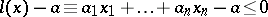(1)

or of the form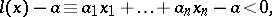(2)

where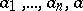are arbitrary real numbers and.

In a wider sense, a linear inequality is an inequality of the form(3)

or of the form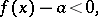(4)

whereis a linear (that is, additive and homogeneous) function on a real vector spacewith values from the fieldof real numbers and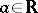. A further generalization of the concept of a linear inequality is obtained if instead ofone takes an arbitrary ordered field. The modern theory of linear inequalities has been constructed on the basis of this generalization (see ).

A number of important questions in analytical mechanics, the geometry of numbers and the approximation of functions reduce to the investigation of systems of linear inequalities. Results concerned with systems of linear inequalities have found very important applications to studies in economics. In such applications, in particular, linear programming did arise. Many practical problems in economic technology and economic planning reduce to the solution of specific systems of linear inequalities; this has significantly determined the modern trend of research in the area of linear inequalities.

In this way there arises, in particular, the main principle of the theory of linear inequalities, the principle of boundary solutions, which was first established (see ) for finite systems of linear inequalities in modulus form, that is, for systems of the form(5)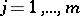, where all theare, in the most general case, elements of the field of complex numbers and all the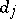are non-negative real numbers,.

The principle of boundary solutions consists of the following. In any compatible system of the form (5) of rankone can select a subsystem of rankconsisting ofinequalities such that at least one solution of the latter, which makes all its inequalities into equalities, satisfies all the inequalities of the system (5), in other words, it is a solution of (5).

The principle of boundary solutions has been extended (see ) to systems of the form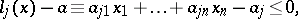(6), over the field(that is, to systems with real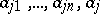,) in the form of the following stronger assertion. In a complete system (6) of rank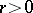one can select a subsystem of rankconsisting ofinequalities such that any solution of the subsystem that makes all its inequalities into equalities satisfies all the inequalities (6) (for a system of the form (6) this assertion turns out to be equivalent to the previous one). The rank of a system of linear inequalities is the largest number of linearly independent formsthat occur in its inequalities.

The principle of boundary solutions has also been extended to systems of the form (6) over an arbitrary ordered fieldand even to more general systems consisting of finitely many linear inequalities of the form (3) over(see ). This principle implies the following compatibility condition for systems of the form (6) over an arbitrary ordered field. A system (6) of rankis compatible if and only if in its coefficient matrix there is a non-zero minorof ordersuch that for the determinants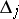,, obtained by bordering it by means of the-th row of this matrix and the column of elements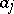, all the ratios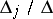are non-negative. In the case of a compatible system of linear equations (cf. Linear equation),, these ratios are zero for any non-zero-th order minorof its coefficient matrix.

The development of the theory of linear inequalities was begun at the end of the 19th century. One of the first propositions of a general character, established in , , was the Minkowski–Farkas theorem, which is now one of the key theorems in the theory of linear inequalities: If all solutions of a compatible system (6) oversatisfy an inequality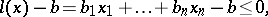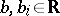,, then there are non-negative numbers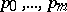such that the identity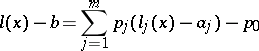holds for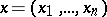.

At the beginning of the 20th century, in the research of G.F. Voronoi devoted to quadratic forms in integer variables, there arose one of the main problems in the theory of linear inequalities, the problem of studying the properties of a convex polyhedron defined in the spaceby the solutions of a compatible finite system of linear inequalities of non-zero rank. The main theorem of Voronoi (see ) expresses conditions such that the polyhedron is non-degenerate, in other words, conditions for the compatibility of a finite system consisting of inequalities of the form (2). For a long time the problem of studying the polyhedron of solutions of systems of the form (6) and the results of H. Minkowski  and J. Farkas  determined the main trend of research on linear inequalities (see ).

Later it became clear how the results of the theory of linear inequalities concerned with finite systems of inequalities of the form (6), particularly the results of Minkowski, Farkas and Voronoi mentioned above, can be derived from the principle of boundary solutions by discrete methods, that is, without using topological properties of the fieldof real numbers (or any consequence of these properties), and the principle of boundary solutions itself was proved by discrete methods (see  and ). Therefore, it turned out to be possible to replace the ground fieldin the construction of the theory of finite systems of linear inequalities by an arbitrary ordered field. Thus the way was prepared for the construction of a purely algebraic theory of linear inequalities, using only discrete methods.

For a long time the theory of linear inequalities did not comprise efficient methods for finding solutions of finite systems of linear inequalities. The method consisting in the direct use of the principle of boundary solutions was not very efficient. Efficient methods for finding individual solutions of a finite system of linear inequalities (in particular, the simplex method) emerged with the rise of linear programming.

In 1960–1965 the so-called method of convolution of systems of linear inequalities was developed, which made it possible to find, from any finite system consisting, in general, of inequalities of the form (3) and (4), particularly of inequalities of the form (1) and (2), and from a given subspace of the space associated with it, a new finite system of linear inequalities whose solution set coincides with some projection of the solution set of the system in question on the chosen subspace (see ). The fundamental convolution algorithm developed on this basis (a special algorithm for the successive elimination of unknowns in the system (6)) makes it possible to obtain general formulas that determine the whole set of solutions of a compatible system of the form (6). The method of convolution can be used in problems of linear programming for reducing the number of unknowns, and also for obtaining general formulas that determine the whole set of optimal solutions (see ). It also has been shown  that by means of the method of convolution it is possible to distinguish maximal compatible subsystems of an incompatible finite system of linear inequalities, which consists in general of inequalities of the form (3) and (4), particularly of inequalities of the form (1) and (2). This makes it possible to use it in one of the approaches to the solution of problems on pattern recognition (see ).

Among infinite systems of linear inequalities a particular class has been distinguished and studied, namely the polyhedrally-closed systems (see ). In the case of the real vector space, polyhedrally-closed systems are defined as infinite systems of the form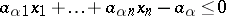,, with a topologically closed conjugate cone in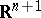, that is, the cone generated by elementswhereis the zero element of. For infinite polyhedrally-closed systems of linear inequalities a number of properties of finite systems of linear inequalities is preserved; in particular, the Minkowski–Farkas theorem holds for such systems. Polyhedrally-closed systems of linear inequalities are used in the theory of approximation of functions, in convex programming and in control theory (see ).

How to Cite This Entry:
Linear inequality. Encyclopedia of Mathematics. URL: http://encyclopediaofmath.org/index.php?title=Linear_inequality&oldid=13735
This article was adapted from an original article by S.N. Chernikov (originator), which appeared in Encyclopedia of Mathematics - ISBN 1402006098. See original article